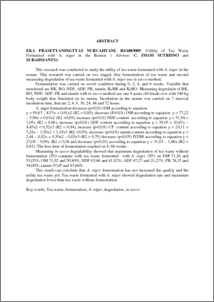# Utillity of Tea Waste Fermented with A. niger in the Rumen

Nurcahyani, Eka Prasetyaningtyas (2005) Utillity of Tea Waste Fermented with A. niger in the Rumen. Masters thesis, University of Diponegoro.Preview
PDF
9Kb

## Abstract

ABSTRACT EKA PRASETYANINGTYAS NURCAHYANI. H4A003005. Utillity of Tea Waste Fermented with A. niger in the Rumen. ( Advisor: C. IMAM SUTRISNO and SURAHMANTO) This research was conducted to study the utility of tea waste fermented with A. niger in the rumen. This research was carried on two staged, first fermentation of tea waste and second measuring degredation of tea waste fermented with A. niger use in sacco method. Fermentation was carried on aerob condition during 0, 2, 4, and 6 weeks. Variable that monitored are BK, BO, NDF, ADF, PK, tannin, KcBK and KcBO. Measuring degredatin of BK, BO, NDF, ADF, PK and tannin with in sacco method use one 6 years old fenale cow with 346 kg body weight that fistulated on its rumen. Incubation in the rumen was carried on 7 interval incubation time, that are 2, 4, 8, 16, 24, 48 and 72 hours. A. niger fermentation decrease (p<0,01) DM according to equation y = 89,63 – 8,53x + 0,91x2 (R2 = 0,83), decrease (P<0,01) OM according to equation y = 77,22 – 5,96x = 0,63x2 (R2 =0,69), increase (p<0.01) NDF content according to equation y = 51,94 = 1,05x (R2 = 0,66), increase (p<0,01) ADF content according to equation y = 38,03 = 10,47x – 4,45x2 = 0,52x3 (R2 = 0,94), increase (p<0,01) CP content according to equation y = 20,11 = 3,28x – 1,50x2 = 1,18x3 (R2 =0,59), decrease (p<0,01) tannin content according to equation y = 2,44 – 0,92x + 0,30x2 – 0,03x3 (R2 = 0,79) decrease (p<0,05) IVDM according to equation y = 27,08 – 0,99x (R2 = 0,38 and decrease (p<0,01) according to equation y = 31,03 – 1,66x (R2 = 0,61). The best time of fermentation reached on 4, 68 weeks. Measuring in sacco degradability showed that maximum degredation of tea waste without fermentation (T0) compare with tea waste fermented with A. niger (TF) on DM 71,26 and 51,05%; OM 71,92 and 50,89%; NDF 63,66 and 41,82%; ADF 47,27 and 21,27%; PK 78,15 and 54,00%; tannin 97,05 and 83,66%. This result can conclude that A. niger fermentation has not increased the quality and the utility tea waste yet. Tea waste fermented with A. niger showed degredation rate and maximum degredation lower than tea waste without fermentation. Key words; Tea waste, fermentation, A. niger, degredation, in sacco

Item Type: Thesis (Masters) Advisor: C. IMAM SUTRISNO and SURAHMANTO Tea waste, fermentation, A. niger, degredation, in sacco S Agriculture > S Agriculture (General) Faculty of Animal and Agricultural Sciences > Department of Animal AgricultureSchool of Postgraduate (mixed) > Master Program in Animal Agriculture 14918 Mr. Magister Ilmu Ternak Admin 19 Jun 2010 10:08 19 Jun 2010 10:08

Repository Staff Only: item control page